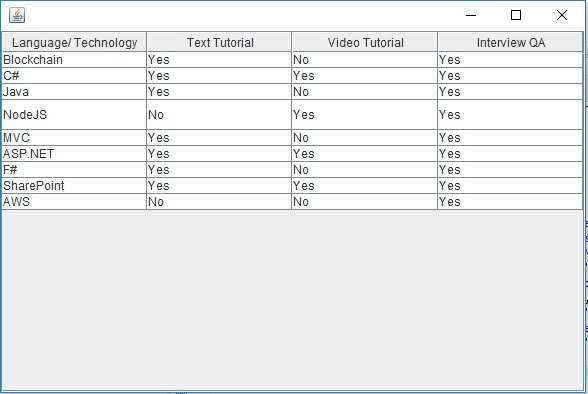# Java Program to set the height of only a single row in a JTable with multiple rows

To set the row height, use the setRowHeight and set a parameter that would be the number of pixels you need to set the row height.

However, if you want to set the row height of a single row then you need to use the same method, but set an additional parameter as shown below −

table.setRowHeight(3, 30);

The above sets row height to 30 pixels for row index 4.

The following is an example to set the height of a row in a table −

## Example

package my;
import javax.swing.JFrame;
import javax.swing.JScrollPane;
import javax.swing.JTable;
import javax.swing.table.DefaultTableModel;
public class SwingDemo {
public static void main(String[] argv) throws Exception {
DefaultTableModel tableModel = new DefaultTableModel();
JTable table = new JTable(tableModel);
tableModel.addRow(new Object[] { "Blockchain", "Yes", "No", "Yes"});
tableModel.addRow(new Object[] { "C#", "Yes", "Yes", "Yes"});
tableModel.addRow(new Object[] { "Java", "Yes", "No", "Yes"});
tableModel.addRow(new Object[] { "NodeJS", "No", "Yes", "Yes"});
tableModel.addRow(new Object[] { "MVC", "Yes", "No", "Yes"});
tableModel.addRow(new Object[] { "ASP.NET", "Yes", "Yes", "Yes"});
tableModel.addRow(new Object[] { "F#", "Yes", "No", "Yes"});
tableModel.addRow(new Object[] { "SharePoint", "Yes", "Yes", "Yes"});
tableModel.addRow(new Object[] { "AWS", "No", "No", "Yes"});
table.setRowHeight(3, 30);
JFrame f = new JFrame();
f.setSize(600, 400);
}